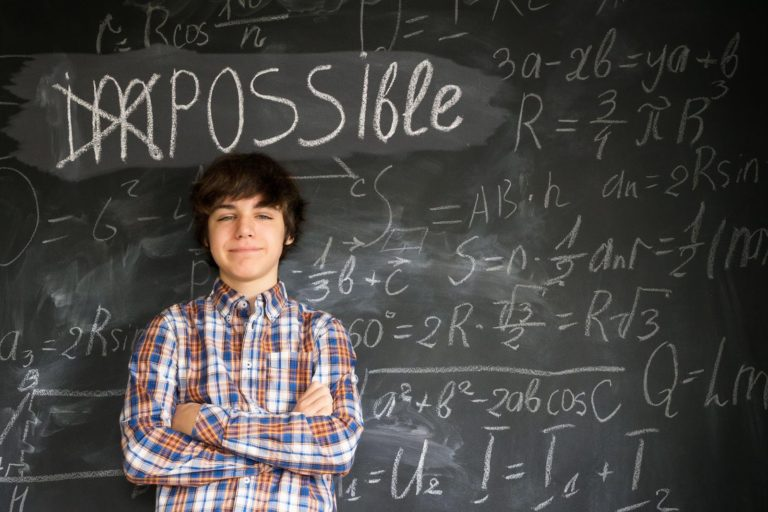## 5 Effective Mental Math Practice Techniques for Young Kids

Mental maths is the ability to perform mathematical calculations in your head without using pen and paper or a calculator. It requires strong memory skills. To retrieve the number combinations and facts requires good long-term memory. Working memory also plays a vital role in tracking the steps needed to solve a particular problem.## The Ultimate Guide to Preparing for a Math Olympiad

Have you ever wondered what it would be like to compete on the international level in maths olympiad?

Want to know more? You are in the right place. Read further to know the details about the Math Olympiad and best practices before going to the olympiad.## How to Quickly Understand Mathematical Ideas in Just Three EASY STEPS!

Mental Mathematics is the brain’s ability to quickly calculate the math problems in mind without using calculator paper and pen.

Unlike traditional math in school, where most students simply opt to memorize the steps. Mental mathematics helps you understand the core of the problem.

This way, you can twist it, break it and reorganize to find the answers.